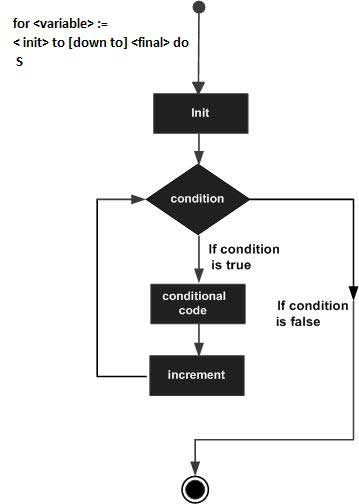### Forum

Wiki## Pascal - For-do Loop

Contents[Hide]

A for-do loop is a repetition control structure that allows you to efficiently write a loop that needs to execute a specific number of times.

## 1. Syntax:

The syntax for the for-do loop in Pascal is as follows:

```for:= to [down to] do
S;
```

Where, the variable-name specifies a variable of ordinal type, called control variable or index variable; initial_value and final_value values are values that the control variable can take; and S is the body of the for-do loop that could be a simple statement or a group of statements.

For example,

```for i:=1 to 10 do writeln(i);
```

Here is the flow of control in a for-do loop:

• The initial step is executed first, and only once. This step allows you to declare and initialize any loop control variables.
• Next, the condition is evaluated. If it is true, the body of the loop is executed. If it is false, the body of the loop does not execute and flow of control jumps to the next statement just after the for-do loop.
• After the body of the for-do loop executes, the value of the variable is either increased or decreased.
• The condition is now evaluated again. If it is true, the loop executes and the process repeats itself (body of loop, then increment step, and then again condition). After the condition becomes false, the for-do loop terminates.

## 2. Flow Diagram## 3. Example:

```program forLoop;
var
a: integer;
begin
for a :=10  to 20 do
begin
writeln('value of a: ', a);
end;
end.
```

When the above code is compiled and executed, it produces following result:

```value of a: 10
value of a: 11
value of a: 12
value of a: 13
value of a: 14
value of a: 15
value of a: 16
value of a: 17
value of a: 18
value of a: 19
value of a: 20

```